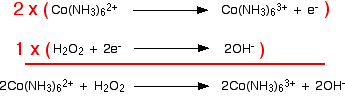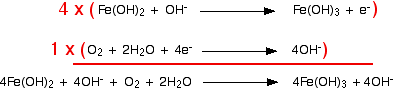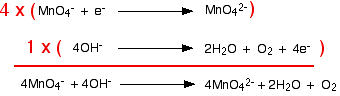Need help? Email help@shout.education

# Writing Ionic Equations for Redox Reactions Done Under Alkaline Conditions

This page explains how to work out electron-half-reactions for oxidation and reduction processes which are carried out under alkaline conditions, and then how to combine them to give the overall ionic equation for the redox reaction. Combining them is easy; working them out may be more difficult than under acidic conditions.

Warning! If you have come to this page directly via a search engine you should be aware that it follows-on from an introductory page on this technique . You would find it much easier to understand the current page if you read that page first.

### Why is it More Difficult to Write Electron Half-equations for These Reactions?

When you are trying to balance electron half-equations, you are only allowed to add:

• electrons
• water
• hydrogen ions (unless the reaction is being done under alkaline conditions – in which case, you can add hydroxide ions instead)

If you are working under acidic or neutral conditions, the sequence is usually:

• Balance the atoms apart from oxygen and hydrogen.
• Balance the oxygens by adding water molecules.
• Balance the hydrogens by adding hydrogen ions.
• Balance the charges by adding electrons.

The whole process is fairly automatic, and provided you take care, there isn't much to go wrong.

#### How is this different under alkaline conditions?

The problem is that the water and the hydroxide ions that you add to balance the equations under alkaline conditions contain both hydrogen and oxygen.

To balance the oxygens, you could in principle add either H2O or OH- to the equation. The same thing is true for balancing the hydrogens. How do you know what to start with?

### How to Tackle the Problem

In some cases, it is obvious how to build up the half-equation using hydroxide ions. Always check this before you get involved in anything more difficult. You will see what I mean shortly.

If it isn't immediately obvious, work out the electron-half equation as if it were being done under acidic conditions just as you have learnt to do on the previous page – in other words by writing in water molecules, hydrogen ions and electrons.

Once you have got a balanced half-equation, you then convert it to alkaline conditions. You will see how to do that in the following examples.

### Four Examples

Don't worry if the chemistry in these examples is unfamiliar to you. It doesn't matter in the slightest. All that matters is how you work out the equations.

#### The oxidation of cobalt(II) to cobalt(III) by hydrogen peroxide

If you add an excess of ammonia solution to a solution containing cobalt(II) ions, you get a complex ion formed called the hexaamminecobalt(II) ion, Co(NH3)62+. This is oxidised rapidly by hydrogen peroxide solution to the hexaamminecobalt(III) ion, Co(NH3)63+.

Ammonia solution is, of course, alkaline.

The half-equation for the cobalt reaction is easy. Start by writing down what you know (or are told):

{\text{Co}(\text{NH}_3)_6}^{2+} \longrightarrow {\text{Co}(\text{NH}_3)_6}^{3+}

Everything balances apart from the charges. Add an electron to the right-hand side to give both sides an overall charge of 2+.

{\text{Co}(\text{NH}_3)_6}^{2+} \longrightarrow {\text{Co}(\text{NH}_3)_6}^{3+} + \color{#467abf}{\text{e}^-}

The hydrogen peroxide half-equation isn't very difficult either, except that you aren't told what is formed and so have to make a guess. It would balance very nicely if you ended up with 2 hydroxide ions on the right-hand side.

This is a good example of a case where it is fairly obvious where to put hydroxide ions.

\text{H}_2\text{O}_2 \longrightarrow 2\text{OH}^-

You would then just have to add 2 electrons to the left-hand side to balance the charges.

\text{H}_2\text{O}_2 + \color{#467abf}{2\text{e}^-} \longrightarrow 2\text{OH}^-

Combining the half-reactions to make the ionic equation for the reaction

What we have so far is:

\begin{aligned} {\text{Co}(\text{NH}_3)_6}^{2+} &\longrightarrow {\text{Co}(\text{NH}_3)_6}^{3+} + \text{e}^- \\ \\ \text{H}_2\text{O}_2 + 2\text{e}^- &\longrightarrow 2\text{OH}^- \end{aligned}

The multiplication and addition looks like this:And that's it – an easy example!

#### The oxidation of iron(II) hydroxide by the air

If you add sodium hydroxide solution to a solution of an iron(II) compound you get a green precipitate of iron(II) hydroxide. This is quite quickly oxidised by oxygen in the air to an orange-brown precipitate of iron(III) hydroxide.

The half-equation for the iron(II) hydroxide is straightforward. Start with what you know:

\text{Fe}(\text{OH})_2 \longrightarrow \text{Fe}(\text{OH})_3

You obviously need another hydroxide ion on the left-hand side. This is even more straightforward than the previous example.

\text{Fe}(\text{OH})_2 + \color{#467abf}{{}^-OH} \longrightarrow \text{Fe}(\text{OH})_3

To balance the charges, add an electron to the right-hand side.

\text{Fe}(\text{OH})_2 + {}^-OH \longrightarrow \text{Fe}(\text{OH})_3 + \color{#467abf}{\text{e}^-}

The half-equation for the oxygen isn't so easy. You don't know what is being formed.

\text{O}_2 \longrightarrow~\color{#467abf}{?}

It isn't at all obvious whether you need to balance the oxygens by adding water molecules or hydroxide ions to the right-hand side. OK – treat it as if it were being done under acidic conditions, and the problem disappears!

In this case, you can only balance the oxygens by adding water molecules to the right-hand side.

\text{O}_2 \longrightarrow \color{#467abf}{2\text{H}_2\text{O}}

Balance the hydrogens by adding hydrogen ions to the left-hand side.

\text{O}_2 + \color{#467abf}{4\text{H}^+} \longrightarrow 2\text{H}_2\text{O}

And then balance the charges by adding 4 electrons:

\text{O}_2 + 4\text{H}^+ + \color{#467abf}{4\text{e}^-} \longrightarrow 2\text{H}_2\text{O}

Now you have got a perfectly balanced half-equation. The problem is, of course, that it only applies under acidic conditions. We should have alkaline conditions – with hydroxide ions present not hydrogen ions.

So get rid of the hydrogen ions! Add enough hydroxide ions to both sides of the equation so that you can neutralise all the hydrogen ions. Because it is now a balanced equation, you must add the same number to both sides to maintain the balance.

\text{O}_2 + 4\text{H}^+ + \color{#467abf}{4\text{OH}^-} + 4\text{e}^- \longrightarrow 2\text{H}_2\text{O} + \color{#467abf}{4\text{OH}^-}

The hydrogen ions and hydroxide ions on the left-hand side would turn into 4 water molecules:

\text{O}_2 + \color{#467abf}{4\text{H}_2\text{O}} + 4\text{e}^- \longrightarrow 2\text{H}_2\text{O} + 4\text{OH}^-

Finally, there are water molecules on both sides of the equation. Cancel out any which aren't changed.

\text{O}_2 + 2\text{H}_2\text{O} + 4\text{e}^- \longrightarrow 4\text{OH}^-

This has all been a bit tedious – although you haven't actually had to think very much! Don't forget to re-check that everything balances now that you have finished.

Combining the half-reactions to make the ionic equation for the reaction

From here on it's all back to the usual routine. We've worked out the two half-equations:

\begin{aligned} \text{Fe}(\text{OH})_2 + {}^-OH &\longrightarrow \text{Fe}(\text{OH})_3 + \text{e}^- \\ \\ \text{O}_2 + 2\text{H}_2\text{O} + 4\text{e}^- &\longrightarrow 4\text{OH}^- \end{aligned}

The iron equation will have to happen 4 times to supply enough electrons to the oxygen.Notice that the hydroxide ions on each side cancel out. (Perhaps to your surprise – certainly to mine when I worked this out!)

4\text{Fe}(\text{OH})_2 + \text{O}_2 + 2\text{H}_2\text{O} \longrightarrow 4\text{Fe}(\text{OH})_3 + \text{e}^-

#### The reduction of manganate(VII) ions to manganate(VI) ions by hydroxide ions

This is a fairly obscure reaction but it is not too difficult to work out and balance. It is unusual in that hydroxide ions are acting as reducing agents.

Dark purple potassium manganate(VII) solution is slowly reduced to dark green potassium manganate(VI) solution by concentrated potassium hydroxide solution. Bubbles of oxygen gas are also given off.

Note: Unless the potassium manganate(VII) solution is very dilute, its strong purple colour tends to mask the green of the potassium manganate(VI) in the short term.

The half-equation for the conversion of manganate(VII) ions to manganate(VI) ions is easy (provided, of course, that you know their formulae!).

{\text{MnO}_4}^- + \text{e}^- \longrightarrow {\text{MnO}_4}^{2-}

So what is happening to the hydroxide ions to turn them into oxygen gas?

It isn't actually very difficult to work out the half-equation directly, but suppose you want to avoid thinking and go through a standard routine:

Write down what you know, balancing the oxygens in the process:

2\text{OH}^- \longrightarrow \text{O}_2

Balance the hydrogens by adding hydrogen ions:

2\text{OH}^- \longrightarrow \color{#467abf}{2\text{H}^+} + \text{O}_2

and now balance the charges:

2\text{OH}^- \longrightarrow 2\text{H}^+ + \text{O}_2 + \color{#467abf}{4\text{e}^-}

Now get rid of the hydrogen ions by adding enough hydroxide ions to both sides of the equation:

2\text{OH}^- + \color{#467abf}{2\text{OH}^-} \longrightarrow 2\text{H}^+ + \color{#467abf}{2\text{OH}^-} + \text{O}_2 + 4\text{e}^-

and tidy it all up!

4\text{OH}^- \longrightarrow \color{#467abf}{2\text{H}_2\text{O}} + \text{O}_2 + 4\text{e}^-

Combining the half-reactions to make the ionic equation for the reaction

What we have so far is:

\begin{aligned} {\text{MnO}_4}^- + \text{e}^- &\longrightarrow {\text{MnO}_4}^{2-} \\ \\ 4\text{OH}^- &\longrightarrow 2\text{H}_2\text{O} + \text{O}_2 + 4\text{e}^- \end{aligned}

The manganese reaction will have to happen four times in order to use up the four electrons produced by the hydroxide equation. Putting this together, you get:The chemistry may be unfamiliar, but working out the equation isn't too hard!

#### The oxidation of chromium(III) to chromium(VI)

If you add an excess of sodium hydroxide solution to a solution containing chromium(III) ions, you get a dark green solution containing the complex ion hexahydroxochromate(III), Cr(OH)63-.

This can be oxidised to bright yellow chromate(VI) ions, CrO42- by warming it with hydrogen peroxide solution.

We've already worked out the hydrogen peroxide half-equation where it is acting as an oxidising agent under alkaline conditions:

\text{H}_2\text{O}_2 + 2\text{e}^- \longrightarrow 2\text{OH}^-

So this time we just need to put together the half-equation for the chromium ions. What we know is:

{\text{Cr}(\text{OH})_6}^{3-} \longrightarrow {\text{CrO}_4}^{2-}

It isn't the least bit obvious where to put hydroxide ions or water molecules, so treat it as if it were being done under acidic conditions. That way, you start by balancing the oxygens by adding water molecules.

To get 6 oxygens on each side, you need two waters on the right-hand side:

{\text{Cr}(\text{OH})_6}^{3-} \longrightarrow {\text{CrO}_4}^{2-} + \color{#467abf}{2\text{H}_2\text{O}}

Now balance the hydrogens by adding hydrogen ions:

{\text{Cr}(\text{OH})_6}^{3-} \longrightarrow {\text{CrO}_4}^{2-} + 2\text{H}_2\text{O} + \color{#467abf}{2\text{H}^+}

and then balance the charges by adding electrons:

{\text{Cr}(\text{OH})_6}^{3-} \longrightarrow {\text{CrO}_4}^{2-} + 2\text{H}_2\text{O} + 2\text{H}^+ + \color{#467abf}{3\text{e}^-}

Finally, convert from acidic to alkaline conditions by adding enough hydroxide ions to both sides to turn the hydrogen ions into water:

{\text{Cr}(\text{OH})_6}^{3-} + \color{#467abf}{2\text{OH}^-} \longrightarrow {\text{CrO}_4}^{2-} + 2\text{H}_2\text{O} + 2\text{H}^+ + \color{#467abf}{2\text{OH}^-} + 3\text{e}^-

and tidy it all up:

{\text{Cr}(\text{OH})_6}^{3-} + 2\text{OH}^- \longrightarrow {\text{CrO}_4}^{2-} + 4\text{H}_2\text{O} + 3\text{e}^-

Combining the half-reactions to make the ionic equation for the reaction

The two half-equations are:

\begin{aligned} \text{H}_2\text{O}_2 + 2\text{e}^- &\longrightarrow 2\text{OH}^- \\ \\ {\text{Cr}(\text{OH})_6}^{3-} + 2\text{OH}^- &\longrightarrow {\text{CrO}_4}^{2-} + 4\text{H}_2\text{O} + 3\text{e}^- \end{aligned}

If you multiply one equation by 3 and the other by 2, that transfers a total of 6 electrons.Finally, tidy up the hydroxide ions that occur on both sides to leave the overall ionic equation:

3\text{H}_2\text{O}_2 + 2{\text{Cr}(\text{OH})_6}^{3-} \longrightarrow 2\text{OH}^- + 2{\text{CrO}_4}^{2-} + 8\text{H}_2\text{O}